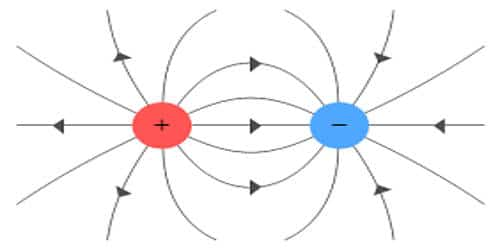# Basic Properties of Electric Charge

Electric Charge is nothing but the amount of energy or electrons that pass from one body to another by different modes like conduction, induction or other specific methods. Charges are present in almost everybody and those bodies which don’t have charges are assumed to be neutrally charged or no charge.

Basic properties of electric charge:

(i) Quantization of electric charge

The fundamental unit of electric charge (e) is the charge carried by the electron and its unit is coulomb has the magnitude 1.6 x 10-19 C. This signifies the fact that charge is a quantized quantity and we can express it as integral multiples of the basic unit of charge (e – charge on one electron). By convention, we take charge of the electron as negative and denote it as “-e” and charge on a proton is simply “e”.

In nature, the electric charge of any system is always an integral multiple of the least amount of charge. It means that the quantity can take only one of the discrete set of values. The charge, q = ne where n is an integer. The basic unit of charge is the charge that an electron or proton carries.

(ii) Conservation of electric charge

Electric charges can neither be created nor destroyed. According to the law of conservation of electric charge, the total charge in an isolated system always remains constant. This implies that charge can neither be created nor be destroyed but can be transferred from one body to another by certain methods like conduction and induction. But the charges can be transferred from one part of the system to another, such that the total charge always remains conserved. For example, Uranium (92U238) can decay by emitting an alpha particle (2He4 nucleus) and transforming it to thorium (90H234).

92U23890H234 + 2He4

Total charge before decay = +92e, total charge after decay = 90e + 2e. Hence, the total charge is conserved. i.e. it remains constant. Here, no charge is created or lost and is only transferred from the one possessing an excess of charge to the other possessing a deficit.

(iii) Additive nature of the charge

The total electric charge of a system is equal to the algebraic sum of electric charges located in the system. This means that they behave like scalars and we can add them directly. For example, if two charged bodies of charges +2q, -5q are brought in contact, the total charge of the system is -3q. These charges have magnitude but no direction, are scalar quantities and are treated like real numbers during conducting any operation.